# Visaton

With 40 years of experience in the field of acoustics and loudspeaker technology Visaton is a future oriented Company. With high engagement in research and development plus the high quality standards of production, Visaton is a specialised and world wide successfully operating German loudspeaker manufacturer.

## Wooferopen a bigger photo

recommended cabinet 1:
closed cabinet with 3,7 L volume
from 66/42 Hz (-3dB/-8dB)

recommended cabinet 2:
14,8 L volume bass reflex cabinet
with HP35 reflex tube, 13 cm long.
from 32/26 Hz (-3dB/-8dB).

## Visaton KT100V 4 Ohm

order no. V-9070

EUR 28,60excl. VAT: € 24.03 / \$ 25.96

9 cm bass driver with high quality cone.

• power handling (continuous/programme) = 25/40 W
• frequency range = 32-9500 Hz
• resonance frequency fs = 37 Hz
• impedance R = 4 Ohm
• sound pressure level SPL = 83 dB (2,83V; 1m)
• DC resistance Re = 3,6 Ohm
• force factor BL = 3,43 N/A
• effective piston radiating area Sd = 54,1 cm2
• effective mechanical mass incl. air load mms = 7,5 g
• equivalent volume of compliance Vas = 9,8 l
• total Q factor Qts = 0,43 (Qms=2,22, Qes=0,54)
• voice coil diameter = 25 mm
• maximum peak linear excursion vibration xlin = +/- 2 mm
• mounting diameter d = 99 mm
• overall diameter d = 94,6 mm
• mounting depth (not countersunk) t = 51,4 mmrecommended cabinet 1:
closed cabinet with 2,7 L volume
from 109/69 Hz (-3dB/-8dB)

recommended cabinet 2:
10,9 L volume bass reflex cabinet
with HP50 reflex tube, 12 cm long.
from 51/43 Hz (-3dB/-8dB).

## Visaton W100S 4 Ohm

order no. V-9020

EUR 35,17excl. VAT: € 29.55 / \$ 31.92

11 cm bass driver with high quality cone.

• power handling (continuous/programme) = 30/50 W
• frequency range = 70-15000 Hz
• resonance frequency fs = 66 Hz
• impedance R = 8 Ohm
• sound pressure level SPL = 92 dB (2,83V; 1m)
• DC resistance Re = 3,3 Ohm
• force factor BL = 3,1 N/A
• voice coil inductance L = 0,5 mH
• effective piston radiating area Sd = 53 cm2
• effective mechanical mass incl. air load mms = 4,2 g
• equivalent volume of compliance Vas = 5,5 l
• total Q factor Qts = 0,49 (Qms=2,45, Qes=0,61)
• voice coil diameter = 20 mm
• maximum peak linear excursion vibration xlin = +/- 2 mm
• mounting diameter d = 94 mm
• overall diameter d = 122 mm
• mounting depth (not countersunk) t = 54 mm## Visaton W100S 8 Ohm

order no. V-9021

EUR 35,17excl. VAT: € 29.55 / \$ 31.92

11 cm bass driver with high quality cone.

• power handling (continuous/programme) = 30/50 W
• frequency range = 70-15000 Hz
• resonance frequency fs = 64 Hz
• impedance R = 8 Ohm
• sound pressure level SPL = 86 dB (2,83V; 1m)
• DC resistance Re = 6,6 Ohm
• force factor BL = 3,9 N/A
• voice coil inductance L = 0,9 mH
• effective piston radiating area Sd = 53 cm2
• effective mechanical mass incl. air load mms = 4,5 g
• equivalent volume of compliance Vas = 5,5 l
• total Q factor Qts = 0,63 (Qms=3,17, Qes=0,78)
• voice coil diameter = 20 mm
• maximum peak linear excursion vibration xlin = +/- 2 mm
• mounting diameter d = 94 mm
• overall diameter d = 122 mm
• mounting depth (not countersunk) t = 54 mmrecommended cabinet 1:
closed cabinet with 1,7 L volume
from 131/83 Hz (-3dB/-8dB)

recommended cabinet 2:
6 L volume bass reflex cabinet
with HP50 reflex tube, 17 cm long.
from 66/53 Hz (-3dB/-8dB).

## Visaton TI100

order no. V-1271

EUR 211,70excl. VAT: € 177.90 / \$ 192.13

11 cm bass driver with high quality cone.

• power handling (continuous/programme) = 40/60 W
• frequency range = 70-20000 Hz
• resonance frequency fs = 62 Hz
• impedance R = 8 Ohm
• sound pressure level SPL = 86 dB (2,83V; 1m)
• DC resistance Re = 6 Ohm
• force factor BL = 4,8 N/A
• voice coil inductance L = 0,7 mH
• effective piston radiating area Sd = 54 cm2
• effective mechanical mass incl. air load mms = 4 g
• equivalent volume of compliance Vas = 6,92 l
• total Q factor Qts = 0,38 (Qms=7,05, Qes=0,4)
• voice coil diameter = 20 mm
• maximum peak linear excursion vibration xlin = +/- 2,3 mm
• mounting diameter d = 100 mm
• overall diameter d = 122 mm
• mounting depth (not countersunk) t = 72 mm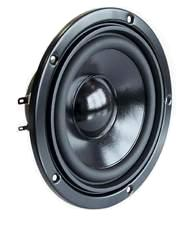recommended cabinet 1:
closed cabinet with 3,9 L volume
from 104/65 Hz (-3dB/-8dB)

recommended cabinet 2:
13,9 L volume bass reflex cabinet
with HP50 reflex tube, 11 cm long.
from 52/42 Hz (-3dB/-8dB).

## Visaton W130S 4 Ohm

order no. V-9022

EUR 36,79excl. VAT: € 30.92 / \$ 33.39

13 cm bass driver with high quality cone.

• power handling (continuous/programme) = 50/80 W
• frequency range = 55-12000 Hz
• resonance frequency fs = 49 Hz
• impedance R = 4 Ohm
• sound pressure level SPL = 90 dB (2,83V; 1m)
• DC resistance Re = 3,7 Ohm
• force factor BL = 3,6 N/A
• voice coil inductance L = 0,55 mH
• effective piston radiating area Sd = 79 cm2
• effective mechanical mass incl. air load mms = 5,4 g
• equivalent volume of compliance Vas = 16 l
• total Q factor Qts = 0,37 (Qms=2,58, Qes=0,43)
• voice coil diameter = 25 mm
• maximum peak linear excursion vibration xlin = +/- 2,3 mm
• mounting diameter d = 108 mm
• overall diameter d = 146 mm
• mounting depth (not countersunk) t = 54 mmrecommended cabinet 1:
closed cabinet with 3,6 L volume
from 106/67 Hz (-3dB/-8dB)

recommended cabinet 2:
12,8 L volume bass reflex cabinet
with HP50 reflex tube, 11 cm long.
from 54/43 Hz (-3dB/-8dB).

## Visaton W130S 8 Ohm

order no. V-9023

EUR 36,79excl. VAT: € 30.92 / \$ 33.39

13 cm bass driver with high quality cone.

• power handling (continuous/programme) = 50/80 W
• frequency range = 55-12000 Hz
• resonance frequency fs = 49 Hz
• impedance R = 8 Ohm
• sound pressure level SPL = 87 dB (2,83V; 1m)
• DC resistance Re = 3,7 Ohm
• force factor BL = 3,6 N/A
• voice coil inductance L = 0,55 mH
• effective piston radiating area Sd = 79 cm2
• effective mechanical mass incl. air load mms = 6,2 g
• equivalent volume of compliance Vas = 16 l
• total Q factor Qts = 0,37 (Qms=2,58, Qes=0,43)
• voice coil diameter = 25 mm
• maximum peak linear excursion vibration xlin = +/- 2,3 mm
• mounting diameter d = 108 mm
• overall diameter d = 146 mm
• mounting depth (not countersunk) t = 54 mm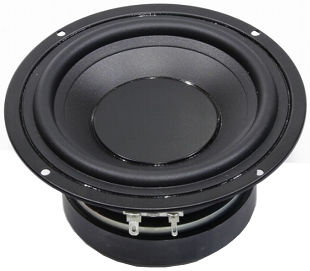open a bigger photo

recommended cabinet 1:
closed cabinet with 1,3 L volume
from 87/55 Hz (-3dB/-8dB)

recommended cabinet 2:
4,5 L volume bass reflex cabinet
with HP35 reflex tube, 27 cm long.
from 44/35 Hz (-3dB/-8dB).

## Visaton W130X 2x4 Ohm

order no. V-9059

EUR 62,09excl. VAT: € 52.18 / \$ 56.35

13 cm bass driver with high quality cone.

• power handling (continuous/programme) = 60/90 W
• frequency range = 40-6000 Hz
• resonance frequency fs = 40 Hz
• impedance R = 2x4 Ohm
• sound pressure level SPL = 78 dB (2,83V; 1m)
• DC resistance Re = 7,9 Ohm
• force factor BL = 13,2 N/A
• voice coil inductance L = 2,75 mH
• effective piston radiating area Sd = 94,2 cm2
• effective mechanical mass incl. air load mms = 37 g
• equivalent volume of compliance Vas = 5,64 l
• total Q factor Qts = 0,37 (Qms=4,39, Qes=0,41)
• voice coil diameter = 40 mm
• maximum peak linear excursion vibration xlin = +/- 5,8 mm
• mounting diameter d = 122 mm
• overall diameter d = 148,2 mm
• mounting depth (not countersunk) t = 72 mm## Visaton WF130ND-8

order no. V-9051

EUR 127,30excl. VAT: € 106.97 / \$ 115.53

12 cm full range driver with polypropylene cone.

• power handling (continuous/programme) = 40/60 W
• frequency range = 46-10000 Hz
• resonance frequency fs = 46 Hz
• impedance R = 8 Ohm
• sound pressure level SPL = 84 dB (2,83V; 1m)
• DC resistance Re = 6,7 Ohm
• force factor BL = 5,39 N/A
• voice coil inductance L = 0,76 mH
• effective piston radiating area Sd = 94 cm2
• effective mechanical mass incl. air load mms = 11,7 g
• equivalent volume of compliance Vas = 10,6 l
• total Q factor Qts = 0,68 (Qms=2,97, Qes=0,89)
• voice coil diameter = 25,6 mm
• maximum peak linear excursion vibration xlin = +/- 1,8 mm
• mounting diameter d = 122,7 mm
• overall diameter d = 123,5 mm
• mounting depth (not countersunk) t = 32,8 mm
• mass m = 0,26 kgrecommended cabinet 1:
closed cabinet with 3,5 L volume
from 87/55 Hz (-3dB/-8dB)

recommended cabinet 2:
12,4 L volume bass reflex cabinet
with HP50 reflex tube, 19 cm long.
from 44/35 Hz (-3dB/-8dB).

## Visaton AL130 8 Ohm

order no. V-1301

EUR 171,30excl. VAT: € 143.95 / \$ 155.47

14 cm bass driver with high quality cone.

• power handling (continuous/programme) = 60/90 W
• frequency range = 50-8000 Hz
• resonance frequency fs = 40 Hz
• impedance R = 8 Ohm
• sound pressure level SPL = 87 dB (2,83V; 1m)
• DC resistance Re = 5,6 Ohm
• force factor BL = 5,64 N/A
• voice coil inductance L = 0,85 mH
• effective piston radiating area Sd = 78,5 cm2
• effective mechanical mass incl. air load mms = 8,8 g
• equivalent volume of compliance Vas = 15,6 l
• total Q factor Qts = 0,36 (Qms=5,19, Qes=0,39)
• voice coil diameter = 25 mm
• maximum peak linear excursion vibration xlin = +/- 6 mm
• mounting diameter d = 114 mm
• overall diameter d = 153 mm
• mounting depth (not countersunk) t = 58 mm

## Visaton W170 8 Ohm

order no. V-9063

EUR 24,39excl. VAT: € 20.50 / \$ 22.14

16 cm bass driver with high quality cone.

• power handling (continuous/programme) = 40/70 W
• frequency range = -6000 Hz
• resonance frequency fs = 57 Hz
• impedance R = 8 Ohm
• sound pressure level SPL = 87 dB (2,83V; 1m)
• DC resistance Re = 7,2 Ohm
• force factor BL = 5,0 N/A
• voice coil inductance L = 0,9 mH
• effective piston radiating area Sd = 137 cm2
• effective mechanical mass incl. air load mms = 8,7 g
• equivalent volume of compliance Vas = 24 l
• total Q factor Qts = 0,69 (Qms=2,92, Qes=0,9)
• voice coil diameter = 25 mm
• maximum peak linear excursion vibration xlin = +/- 7,5 mm
• mounting diameter d = 146 mm
• overall diameter d = 170 mm
• mounting depth (not countersunk) t = 73 mm
• mass m = 0,77 kgopen a bigger photo

recommended cabinet 1:
closed cabinet with 12,7 L volume
from 67/43 Hz (-3dB/-8dB)

recommended cabinet 2:
49 L volume bass reflex cabinet
with HP70 reflex tube, 13 cm long.
from 33/27 Hz (-3dB/-8dB).

## Visaton W170S 4 Ohm

order no. V-9024

EUR 46,67excl. VAT: € 39.22 / \$ 42.36

17 cm bass driver with paper cone. The diaphragm is varnished with a hydrophabic coat.

• power handling (continuous/programme) = 50/80 W
• frequency range = -8000 Hz
• resonance frequency fs = 36 Hz
• impedance R = 4 Ohm
• sound pressure level SPL = 89 dB (2,83V; 1m)
• DC resistance Re = 3,2 Ohm
• force factor BL = 4,4 N/A
• voice coil inductance L = 0,6 mH
• effective piston radiating area Sd = 129 cm2
• effective mechanical mass incl. air load mms = 12 g
• equivalent volume of compliance Vas = 38 l
• total Q factor Qts = 0,41 (Qms=2,16, Qes=0,51)
• voice coil diameter = 25 mm
• maximum peak linear excursion vibration xlin = +/- 10 mm
• mounting diameter d = 148 mm
• overall diameter d = 187 mm
• mounting depth (not countersunk) t = 81 mm
• mass m = 1,1 kgopen a bigger photo

recommended cabinet 1:
closed cabinet with 22 L volume
from 56/35 Hz (-3dB/-8dB)

recommended cabinet 2:
92 L volume bass reflex cabinet
with HP70 reflex tube, 9 cm long.
from 26/22 Hz (-3dB/-8dB).

## Visaton W170S 8 Ohm

order no. V-9025

EUR 46,67excl. VAT: € 39.22 / \$ 42.36

17 cm bass driver with paper cone. The diaphragm is varnished with a hydrophabic coat.

• power handling (continuous/programme) = 50/80 W
• frequency range = -8000 Hz
• resonance frequency fs = 36 Hz
• impedance R = 8 Ohm
• sound pressure level SPL = 86 dB (2,83V; 1m)
• DC resistance Re = 5,9 Ohm
• force factor BL = 5,4 N/A
• voice coil inductance L = 1,2 mH
• effective piston radiating area Sd = 129 cm2
• effective mechanical mass incl. air load mms = 13 g
• equivalent volume of compliance Vas = 38 l
• total Q factor Qts = 0,52 (Qms=2,43, Qes=0,66)
• voice coil diameter = 25 mm
• maximum peak linear excursion vibration xlin = +/- 10 mm
• mounting diameter d = 148 mm
• overall diameter d = 187 mm
• mounting depth (not countersunk) t = 81 mm
• mass m = 1,1 kg

## Visaton WS17E 4 Ohm

order no. V-1054

EUR 29,94excl. VAT: € 25.16 / \$ 27.17

17 cm bass driver with paper cone. The diaphragm is varnished with a hydrophabic coat.

• power handling (continuous/programme) = 60/90 W
• frequency range = -7000 Hz
• resonance frequency fs = 45 Hz
• impedance R = 4 Ohm
• sound pressure level SPL = 91 dB (2,83V; 1m)
• DC resistance Re = 3,2 Ohm
• force factor BL = 3,9 N/A
• voice coil inductance L = 0,6 mH
• effective piston radiating area Sd = 143 cm2
• effective mechanical mass incl. air load mms = 16 g
• equivalent volume of compliance Vas = 22 l
• total Q factor Qts = 0,65 (Qms=2,35, Qes=0,9)
• voice coil diameter = 25 mm
• maximum peak linear excursion vibration xlin = +/- 6,5 mm
• mounting diameter d = 150 mm
• overall diameter d = 188 mm
• mounting depth (not countersunk) t = 79 mm
• mass m = 0,97 kg

## Visaton WS17E 8 Ohm

order no. V-1055

EUR 29,94excl. VAT: € 25.16 / \$ 27.17

17 cm bass driver with paper cone. The diaphragm is varnished with a hydrophabic coat.

• power handling (continuous/programme) = 60/90 W
• frequency range = -7000 Hz
• resonance frequency fs = 45 Hz
• impedance R = 8 Ohm
• sound pressure level SPL = 88 dB (2,83V; 1m)
• DC resistance Re = 5,8 Ohm
• force factor BL = 3,9 N/A
• voice coil inductance L = 0,9 mH
• effective piston radiating area Sd = 143 cm2
• effective mechanical mass incl. air load mms = 16 g
• equivalent volume of compliance Vas = 22 l
• total Q factor Qts = 0,81 (Qms=2,2, Qes=1,28)
• voice coil diameter = 25 mm
• maximum peak linear excursion vibration xlin = +/- 6,5 mm
• mounting diameter d = 150 mm
• overall diameter d = 188 mm
• mounting depth (not countersunk) t = 79 mm
• mass m = 0,97 kg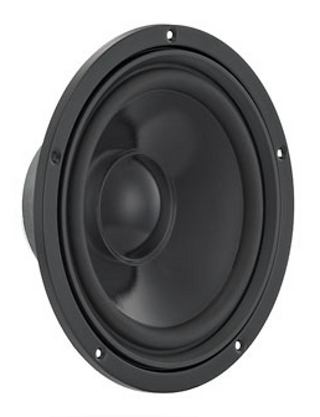open a bigger photo

recommended cabinet 1:
closed cabinet with 13,7 L volume
from 65/41 Hz (-3dB/-8dB)

recommended cabinet 2:
47 L volume bass reflex cabinet
with HP70 reflex tube, 16 cm long.
from 33/26 Hz (-3dB/-8dB).

## Visaton W200S 4 Ohm

order no. V-9029

EUR 68,06excl. VAT: € 57.19 / \$ 61.77

21 cm bass driver with paper cone. The diaphragm is varnished with a hydrophabic coat.

• power handling (continuous/programme) = 75/115 W
• frequency range = -6000 Hz
• resonance frequency fs = 29 Hz
• impedance R = 4 Ohm
• sound pressure level SPL = 91 dB (2,83V; 1m)
• DC resistance Re = 3,4 Ohm
• force factor BL = 7,7 N/A
• voice coil inductance L = 1,5 mH
• effective piston radiating area Sd = 214 cm2
• effective mechanical mass incl. air load mms = 28 g
• equivalent volume of compliance Vas = 65 l
• total Q factor Qts = 0,35 (Qms=3,99, Qes=0,38)
• voice coil diameter = 35 mm
• maximum peak linear excursion vibration xlin = +/- 10 mm
• mounting diameter d = 184 mm
• overall diameter d = 232 mm
• mounting depth (not countersunk) t = 95 mm
• mass m = 1,5 kg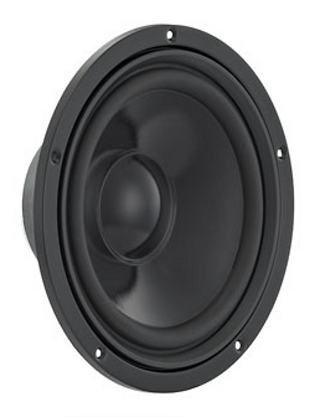open a bigger photo

recommended cabinet 1:
closed cabinet with 12 L volume
from 73/46 Hz (-3dB/-8dB)

recommended cabinet 2:
39 L volume bass reflex cabinet
with HP70 reflex tube, 15 cm long.
from 39/30 Hz (-3dB/-8dB).

## Visaton W200S 8 Ohm

order no. V-9030

EUR 68,06excl. VAT: € 57.19 / \$ 61.77

21 cm bass driver with paper cone. The diaphragm is varnished with a hydrophabic coat.

• power handling (continuous/programme) = 75/115 W
• frequency range = -6000 Hz
• resonance frequency fs = 30 Hz
• impedance R = 8 Ohm
• sound pressure level SPL = 88 dB (2,83V; 1m)
• DC resistance Re = 6,0 Ohm
• force factor BL = 9,0 N/A
• voice coil inductance L = 2,2 mH
• effective piston radiating area Sd = 214 cm2
• effective mechanical mass incl. air load mms = 26 g
• equivalent volume of compliance Vas = 70 l
• total Q factor Qts = 0,33 (Qms=3,59, Qes=0,36)
• voice coil diameter = 35 mm
• maximum peak linear excursion vibration xlin = +/- 10 mm
• mounting diameter d = 184 mm
• overall diameter d = 232 mm
• mounting depth (not countersunk) t = 95 mm
• mass m = 1,5 kg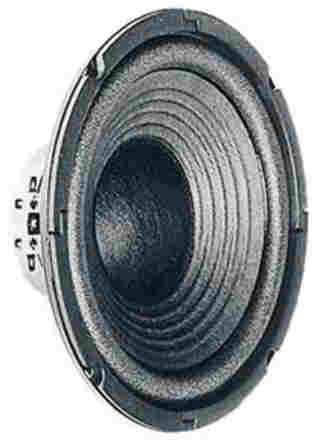open a bigger photo

recommended cabinet 1:
closed cabinet with 28 L volume
from 75/47 Hz (-3dB/-8dB)

recommended cabinet 2:
116 L volume bass reflex cabinet
with 4x HP100 reflex tube, 39 cm long.
from 34/29 Hz (-3dB/-8dB).

## Visaton W200 4 Ohm

order no. V-9064

EUR 34,44excl. VAT: € 28.94 / \$ 31.26

19 cm bass driver with high quality cone.

• power handling (continuous/programme) = 50/80 W
• frequency range = -8000 Hz
• resonance frequency fs = 49 Hz
• impedance R = 4 Ohm
• sound pressure level SPL = 91 dB (2,83V; 1m)
• DC resistance Re = 3,2 Ohm
• force factor BL = 4,0 N/A
• voice coil inductance L = 0,5 mH
• effective piston radiating area Sd = 207 cm2
• effective mechanical mass incl. air load mms = 14 g
• equivalent volume of compliance Vas = 45 l
• total Q factor Qts = 0,51 (Qms=2,81, Qes=0,62)
• voice coil diameter = 25 mm
• maximum peak linear excursion vibration xlin = +/- 10 mm
• mounting diameter d = 185 mm
• overall diameter d = 206 mm
• mounting depth (not countersunk) t = 87 mm
• mass m = 1045 kg

## Visaton W200 8 Ohm

order no. V-9065

EUR 34,44excl. VAT: € 28.94 / \$ 31.26

19 cm bass driver with high quality cone.

• power handling (continuous/programme) = 80/80 W
• frequency range = -8000 Hz
• resonance frequency fs = 46 Hz
• impedance R = 8 Ohm
• sound pressure level SPL = 88 dB (2,83V; 1m)
• DC resistance Re = 6,8 Ohm
• force factor BL = 5,6 N/A
• voice coil inductance L = 0,9 mH
• effective piston radiating area Sd = 207 cm2
• effective mechanical mass incl. air load mms = 14 g
• equivalent volume of compliance Vas = 51 l
• total Q factor Qts = 0,62 (Qms=2,82, Qes=0,80)
• voice coil diameter = 25 mm
• maximum peak linear excursion vibration xlin = +/- 10 mm
• mounting diameter d = 185 mm
• overall diameter d = 206 mm
• mounting depth (not countersunk) t = 87 mm
• mass m = 1045 kg

## Visaton WS20E 4 Ohm

order no. V-1057

EUR 36,22excl. VAT: € 30.44 / \$ 32.87

21 cm bass driver with polyethylene cone.

• power handling (continuous/programme) = 80/120 W
• frequency range = -7000 Hz
• resonance frequency fs = 47 Hz
• impedance R = 4 Ohm
• sound pressure level SPL = 91 dB (2,83V; 1m)
• DC resistance Re = 3,3 Ohm
• force factor BL = 4,5 N/A
• voice coil inductance L = 0,7 mH
• effective piston radiating area Sd = 240 cm2
• effective mechanical mass incl. air load mms = 23 g
• equivalent volume of compliance Vas = 39 l
• total Q factor Qts = 1,14 (Qms=5,56, Qes=1,44)
• voice coil diameter = 30 mm
• maximum peak linear excursion vibration xlin = +/- 6 mm
• mounting diameter d = 186 mm
• overall diameter d = 236 mm
• mounting depth (not countersunk) t = 85,9 mm
• mass m = 1,3 kg

## Visaton WS20E 8 Ohm

order no. V-1056

EUR 36,22excl. VAT: € 30.44 / \$ 32.87

21 cm bass driver with polypropylene cone.

• power handling (continuous/programme) = 80/120 W
• frequency range = -7000 Hz
• resonance frequency fs = 47 Hz
• impedance R = 8 Ohm
• sound pressure level SPL = 88 dB (2,83V; 1m)
• DC resistance Re = 6,8 Ohm
• force factor BL = 5,5 N/A
• voice coil inductance L = 1,0 mH
• effective piston radiating area Sd = 240 cm2
• effective mechanical mass incl. air load mms = 21 g
• equivalent volume of compliance Vas = 44 l
• total Q factor Qts = 1,23 (Qms=5,36, Qes=1,59)
• voice coil diameter = 30 mm
• maximum peak linear excursion vibration xlin = +/- 6 mm
• mounting diameter d = 186 mm
• overall diameter d = 236 mm
• mounting depth (not countersunk) t = 85,9 mm
• mass m = 1,3 kgrecommended cabinet 1:
closed cabinet with 12,3 L volume
from 80/50 Hz (-3dB/-8dB)

recommended cabinet 2:
38 L volume bass reflex cabinet
with HP100 reflex tube, 30 cm long.
from 43/33 Hz (-3dB/-8dB).

## Visaton AL 200 8 Ohm

order no. V-1281

EUR 211,40excl. VAT: € 177.65 / \$ 191.86

21 cm bass driver with high quality cone.

• power handling (continuous/programme) = 120/180 W
• frequency range = 40-9000 Hz
• resonance frequency fs = 32 Hz
• impedance R = 8 Ohm
• sound pressure level SPL = 88 dB (2,83V; 1m)
• DC resistance Re = 6,7 Ohm
• force factor BL = 8,82 N/A
• voice coil inductance L = 1,2 mH
• effective piston radiating area Sd = 216 cm2
• effective mechanical mass incl. air load mms = 21 g
• equivalent volume of compliance Vas = 77 l
• total Q factor Qts = 0,32 (Qms=3,92, Qes=0,35)
• voice coil diameter = 38 mm
• maximum peak linear excursion vibration xlin = +/- 7 mm
• mounting diameter d = 189 mm
• overall diameter d = 222 mm
• mounting depth (not countersunk) t = 90 mm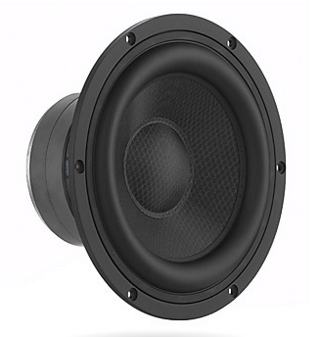open a bigger photo

recommended cabinet 1:
closed cabinet with 21 L volume
from 53/33 Hz (-3dB/-8dB)

recommended cabinet 2:
83 L volume bass reflex cabinet
with HP100 reflex tube, 31 cm long.
from 25/21 Hz (-3dB/-8dB).

## Visaton TIW200XS

order no. V-1340

EUR 188,00excl. VAT: € 157.98 / \$ 170.62

21 cm bass driver with sandwich cone. The basket is made of die-cast. The voice coil is ventilated.

• power handling (continuous/programme) = 120/180 W
• frequency range = -7500 Hz
• resonance frequency fs = 30 Hz
• impedance R = 8 Ohm
• sound pressure level SPL = 84 dB (2,83V; 1m)
• DC resistance Re = 6,0 Ohm
• force factor BL = 8,51 N/A
• voice coil inductance L = 1,6 mH
• effective piston radiating area Sd = 206 cm2
• effective mechanical mass incl. air load mms = 33 g
• equivalent volume of compliance Vas = 51,5 l
• total Q factor Qts = 0,46 (Qms=5,19, Qes=0,5)
• voice coil diameter = 38 mm
• maximum peak linear excursion vibration xlin = +/- 12,5 mm
• mounting diameter d = 190 mm
• overall diameter d = 222 mm
• mounting depth (not countersunk) t = 112 mm
• mass m = 3,5 kg

## Visaton GF200 (one coil)

order no. V-1330

EUR 167,50excl. VAT: € 140.76 / \$ 152.02

21 cm bass driver with high quality cone. The basket is made of die-cast. The spider is rear ventilated for better cooling.

• power handling (continuous/programme) = 120/180 W
• frequency range = -8000 Hz
• resonance frequency fs = 34 Hz
• impedance R = 4 Ohm
• sound pressure level SPL = 85 dB (2,83V; 1m)
• DC resistance Re = 2,9 Ohm
• force factor BL = 4,6 N/A
• voice coil inductance L = 0,4 mH
• effective piston radiating area Sd = 214 cm2
• effective mechanical mass incl. air load mms = 25 g
• equivalent volume of compliance Vas = 62 l
• total Q factor Qts = 0,77 (Qms=4,51, Qes=0,93)
• voice coil diameter = 38 mm
• maximum peak linear excursion vibration xlin = +/- 10 mm
• mounting diameter d = 190 mm
• overall diameter d = 222 mm
• mounting depth (not countersunk) t = 96 mm
• mass m = 2,5 kgopen a bigger photo

recommended cabinet 1:
closed cabinet with 12,5 L volume
from 71/45 Hz (-3dB/-8dB)

recommended cabinet 2:
41 L volume bass reflex cabinet
with HP70 reflex tube, 15 cm long.
from 37/29 Hz (-3dB/-8dB).

## Visaton GF200

order no. V-1330

EUR 167,50excl. VAT: € 140.76 / \$ 152.02

21 cm bass driver with high quality cone. The basket is made of die-cast. The spider is rear ventilated for better cooling.

• power handling (continuous/programme) = 120/180 W
• frequency range = -8000 Hz
• resonance frequency fs = 30 Hz
• impedance R = 8 Ohm
• sound pressure level SPL = 88 dB (2,83V; 1m)
• DC resistance Re = 5,8 Ohm
• force factor BL = 9,2 N/A
• voice coil inductance L = 1,1 mH
• effective piston radiating area Sd = 214 cm2
• effective mechanical mass incl. air load mms = 25 g
• equivalent volume of compliance Vas = 68 l
• total Q factor Qts = 0,34 (Qms=4,12, Qes=0,37)
• voice coil diameter = 38 mm
• maximum peak linear excursion vibration xlin = +/- 10 mm
• mounting diameter d = 190 mm
• overall diameter d = 222 mm
• mounting depth (not countersunk) t = 96 mm
• mass m = 2,5 kg

## Visaton WS25E

order no. V-1061

EUR 45,77excl. VAT: € 38.46 / \$ 41.54

26 cm bass driver with paper cone. The diaphragm is varnished with a hydrophabic coat.

• power handling (continuous/programme) = 80/110 W
• frequency range = -6000 Hz
• resonance frequency fs = 34 Hz
• impedance R = 8 Ohm
• sound pressure level SPL = 88 dB (2,83V; 1m)
• DC resistance Re = 5,9 Ohm
• force factor BL = 3,8 N/A
• voice coil inductance L = 0,8 mH
• effective piston radiating area Sd = 346 cm2
• effective mechanical mass incl. air load mms = 33 g
• equivalent volume of compliance Vas = 113 l
• total Q factor Qts = 1,43 (Qms=2,82, Qes=2,92)
• voice coil diameter = 25 mm
• maximum peak linear excursion vibration xlin = +/- 6 mm
• mounting diameter d = 225 mm
• overall diameter d = 283 mm
• mounting depth (not countersunk) t = 107 mm
• mass m = 1,25 kg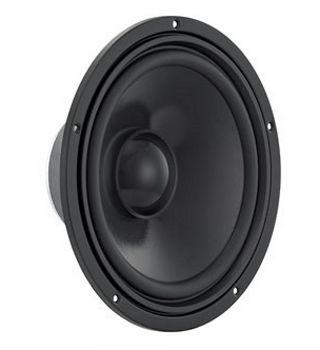open a bigger photo

recommended cabinet 1:
closed cabinet with 30 L volume
from 72/45 Hz (-3dB/-8dB)

recommended cabinet 2:
108 L volume bass reflex cabinet
with HP100 reflex tube, 9 cm long.
from 37/29 Hz (-3dB/-8dB).

## Visaton W250S 4 Ohm

order no. V-9034

EUR 98,61excl. VAT: € 82.87 / \$ 89.49

26 cm bass driver with paper cone. The diaphragm is varnished with a hydrophabic coat.

• power handling (continuous/programme) = 100/150 W
• frequency range = -5500 Hz
• resonance frequency fs = 33 Hz
• impedance R = 4 Ohm
• sound pressure level SPL = 93 dB (2,83V; 1m)
• DC resistance Re = 3,6 Ohm
• force factor BL = 8,94 N/A
• voice coil inductance L = 0,93 mH
• effective piston radiating area Sd = 346 cm2
• effective mechanical mass incl. air load mms = 32 g
• equivalent volume of compliance Vas = 135 l
• total Q factor Qts = 0,35 (Qms=2,01, Qes=0,43)
• voice coil diameter = 38 mm
• maximum peak linear excursion vibration xlin = +/- 7 mm
• mounting diameter d = 233 mm
• overall diameter d = 283 mm
• mounting depth (not countersunk) t = 120 mm
• mass m = 3,0 kgopen a bigger photo

recommended cabinet 1:
closed cabinet with 19 L volume
from 77/49 Hz (-3dB/-8dB)

recommended cabinet 2:
60 L volume bass reflex cabinet
with HP100 reflex tube, 18 cm long.
from 42/32 Hz (-3dB/-8dB).

## Visaton W250S 8 Ohm

order no. V-9035

EUR 98,61excl. VAT: € 82.87 / \$ 89.49

26 cm bass driver with paper cone. The diaphragm is varnished with a hydrophabic coat.

• power handling (continuous/programme) = 100/150 W
• frequency range = -5500 Hz
• resonance frequency fs = 31 Hz
• impedance R = 8 Ohm
• sound pressure level SPL = 90 dB (2,83V; 1m)
• DC resistance Re = 6,6 Ohm
• force factor BL = 9,2 N/A
• voice coil inductance L = 1,5 mH
• effective piston radiating area Sd = 345 cm2
• effective mechanical mass incl. air load mms = 38 g
• equivalent volume of compliance Vas = 121 l
• total Q factor Qts = 0,32 (Qms=1,88, Qes=0,39)
• voice coil diameter = 38 mm
• maximum peak linear excursion vibration xlin = +/- 7 mm
• mounting diameter d = 233 mm
• overall diameter d = 283 mm
• mounting depth (not countersunk) t = 120 mm
• mass m = 3,0 kgopen a bigger photo

recommended cabinet 1:
closed cabinet with 11,3 L volume
from 80/50 Hz (-3dB/-8dB)

recommended cabinet 2:
36 L volume bass reflex cabinet
with HP100 reflex tube, 31 cm long.
from 43/33 Hz (-3dB/-8dB).

## Visaton TIW250XS 8 Ohm

order no. V-1342

EUR 320,20excl. VAT: € 269.08 / \$ 290.60

25 cm bass driver with sandwich cone.

• power handling (continuous/programme) = 200/300 W
• frequency range = -3000 Hz
• resonance frequency fs = 33 Hz
• impedance R = 8 Ohm
• sound pressure level SPL = 89 dB (2,83V; 1m)
• DC resistance Re = 5,6 Ohm
• force factor BL = 13 N/A
• voice coil inductance L = 1,7 mH
• effective piston radiating area Sd = 314 cm2
• effective mechanical mass incl. air load mms = 49 g
• equivalent volume of compliance Vas = 66 l
• total Q factor Qts = 0,33 (Qms=5,21, Qes=0,35)
• voice coil diameter = 50 mm
• maximum peak linear excursion vibration xlin = +/- 14 mm
• mounting diameter d = 235 mm
• overall diameter d = 264 mm
• mounting depth (not countersunk) t = 121,2 mm
• mass m = 5,3 kg

## Visaton W250

order no. V-9067

EUR 56,87excl. VAT: € 47.79 / \$ 51.61

24 cm bass driver with high quality cone.

• power handling (continuous/programme) = 90/130 W
• frequency range = -6000 Hz
• resonance frequency fs = 37 Hz
• impedance R = 8 Ohm
• sound pressure level SPL = 90 dB (2,83V; 1m)
• DC resistance Re = 6,8 Ohm
• force factor BL = 6,4 N/A
• voice coil inductance L = 1,1 mH
• effective piston radiating area Sd = 360 cm2
• effective mechanical mass incl. air load mms = 27 g
• equivalent volume of compliance Vas = 135 l
• total Q factor Qts = 0,78 (Qms=3,57, Qes=1,0)
• voice coil diameter = 35 mm
• maximum peak linear excursion vibration xlin = +/- 10 mm
• mounting diameter d = 236 mm
• overall diameter d = 255 mm
• mounting depth (not countersunk) t = 110 mm
• mass m = 1,5 kgopen a bigger photo

recommended cabinet 1:
closed cabinet with 146 L volume
from 46/29 Hz (-3dB/-8dB)

recommended cabinet 2:
585 L volume bass reflex cabinet
with 2x HP100 reflex tube, 3 cm long.
from 21/18 Hz (-3dB/-8dB).

## Visaton W300 8 Ohm

order no. V-9069

EUR 96,53excl. VAT: € 81.12 / \$ 87.61

29 cm bass driver with high quality cone.

• power handling (continuous/programme) = 120/180 W
• frequency range = -2500 Hz
• resonance frequency fs = 32 Hz
• impedance R = 8 Ohm
• sound pressure level SPL = 91 dB (2,83V; 1m)
• DC resistance Re = 6,9 Ohm
• force factor BL = 9,44 N/A
• voice coil inductance L = 1,77 mH
• effective piston radiating area Sd = 490 cm2
• effective mechanical mass incl. air load mms = 43 g
• equivalent volume of compliance Vas = 190 l
• total Q factor Qts = 0,55 (Qms=2,85, Qes=0,68)
• voice coil diameter = 50 mm
• maximum peak linear excursion vibration xlin = +/- 14 mm
• mounting diameter d = 280 mm
• overall diameter d = 305 mm
• mounting depth (not countersunk) t = 132 mm
• mass m = 2,8 kg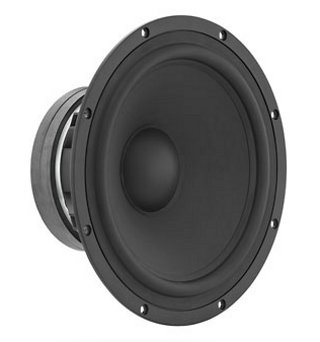open a bigger photo

recommended cabinet 1:
closed cabinet with 19 L volume
from 71/45 Hz (-3dB/-8dB)

recommended cabinet 2:
52 L volume bass reflex cabinet
with HP100 reflex tube, 28 cm long.
from 40/31 Hz (-3dB/-8dB).

## Visaton TIW300 8 Ohm

order no. V-1364

EUR 428,80excl. VAT: € 360.34 / \$ 389.16

31 cm bass driver with high quality cone. The voice coil is ventilated.

• power handling (continuous/programme) = 300/600 W
• frequency range = -4000 Hz
• resonance frequency fs = 25 Hz
• impedance R = 8 Ohm
• sound pressure level SPL = 90 dB (2,83V; 1m)
• DC resistance Re = 5,4 Ohm
• force factor BL = 16,5 N/A
• voice coil inductance L = 1,7 mH
• effective piston radiating area Sd = 510 cm2
• effective mechanical mass incl. air load mms = 92 g
• equivalent volume of compliance Vas = 160 l
• total Q factor Qts = 0,28 (Qms=4,59, Qes=0,30)
• voice coil diameter = 65 mm
• maximum peak linear excursion vibration xlin = +/- 16 mm
• mounting diameter d = 288 mm
• overall diameter d = 329 mm
• mounting depth (not countersunk) t = 149 mm
• mass m = 7,0 kg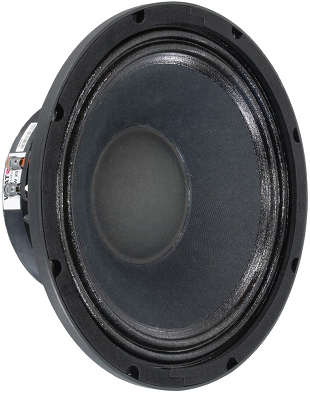open a bigger photo

recommended cabinet 1:
closed cabinet with 7,5 L volume
from 126/80 Hz (-3dB/-8dB)

recommended cabinet 2:
24 L volume bass reflex cabinet
with HP100 reflex tube, 16 cm long.
from 67/52 Hz (-3dB/-8dB).

## Visaton PAW 25

order no. V-3050

EUR 118,80excl. VAT: € 99.83 / \$ 107.82

25 cm bass driver with paper cone. The basket is made of die-cast.

• power handling (continuous/programme) = 300/450 W
• frequency range = -4200 Hz
• resonance frequency fs = 52 Hz
• impedance R = 8 Ohm
• sound pressure level SPL = 92 dB (2,83V; 1m)
• DC resistance Re = 6,5 Ohm
• force factor BL = 15,6 N/A
• voice coil inductance L = 0,9 mH
• effective piston radiating area Sd = 353 cm2
• effective mechanical mass incl. air load mms = 37,6 g
• equivalent volume of compliance Vas = 44 l
• total Q factor Qts = 0,33 (Qms=9,59, Qes=0,34)
• voice coil diameter = 65 mm
• maximum peak linear excursion vibration xlin = +/- 3,5 mm
• mounting diameter d = 229 mm
• overall diameter d = 264 mm
• mounting depth (not countersunk) t = 107 mm
• mass m = 3,61 kg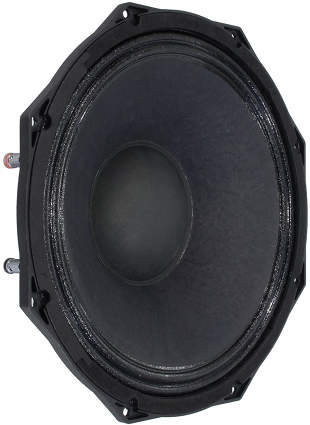open a bigger photo

recommended cabinet 1:
closed cabinet with 16 L volume
from 100/63 Hz (-3dB/-8dB)

recommended cabinet 2:
57 L volume bass reflex cabinet
with 2x HP100 reflex tube, 20 cm long.
from 52/41 Hz (-3dB/-8dB).

## Visaton PAW 30 ND

order no. V-3052

EUR 317,90excl. VAT: € 267.14 / \$ 288.51

31 cm bass driver with paper cone. The basket is made of die-cast. The magnet system is based on Neodymium technology.

• power handling (continuous/programme) = 400/600 W
• frequency range = -5500 Hz
• resonance frequency fs = 45 Hz
• impedance R = 8 Ohm
• sound pressure level SPL = 93 dB (2,83V; 1m)
• DC resistance Re = 6,1 Ohm
• force factor BL = 17,6 N/A
• voice coil inductance L = 0,55 mH
• effective piston radiating area Sd = 539 cm2
• effective mechanical mass incl. air load mms = 69 g
• equivalent volume of compliance Vas = 78 l
• total Q factor Qts = 0,35 (Qms=7,63, Qes=0,37)
• voice coil diameter = 75 mm
• maximum peak linear excursion vibration xlin = +/- 4 mm
• mounting diameter d = 284 mm
• overall diameter d = 329 mm
• mounting depth (not countersunk) t = 132 mm
• mass m = 3,05 kg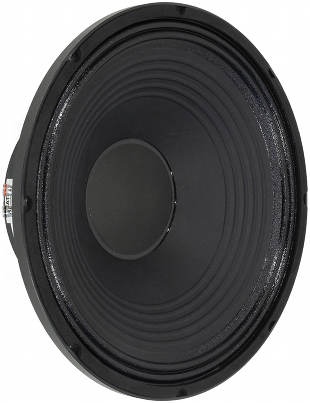open a bigger photo

recommended cabinet 1:
closed cabinet with 27 L volume
from 96/60 Hz (-3dB/-8dB)

recommended cabinet 2:
79 L volume bass reflex cabinet
with 2x HP100 reflex tube, 14 cm long.
from 53/41 Hz (-3dB/-8dB).

## Visaton PAW 38

order no. V-3054

EUR 315,40excl. VAT: € 265.04 / \$ 286.25

38 cm bass driver with paper cone. The basket is made of die-cast.

• power handling (continuous/programme) = 700/1000 W
• frequency range = -3700 Hz
• resonance frequency fs = 36 Hz
• impedance R = 8 Ohm
• sound pressure level SPL = 92 dB (2,83V; 1m)
• DC resistance Re = 7,2 Ohm
• force factor BL = 25 N/A
• voice coil inductance L = 2,6 mH
• effective piston radiating area Sd = 898 cm2
• effective mechanical mass incl. air load mms = 125 g
• equivalent volume of compliance Vas = 195 l
• total Q factor Qts = 0,3 (Qms=9, Qes=0,31)
• voice coil diameter = 100 mm
• maximum peak linear excursion vibration xlin = +/- 5,5 mm
• mounting diameter d = 356 mm
• overall diameter d = 392 mm
• mounting depth (not countersunk) t = 144 mm
• mass m = 11,5 kg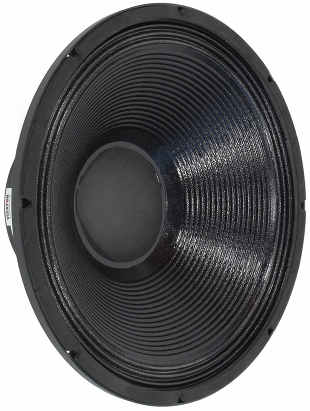open a bigger photo

recommended cabinet 1:
closed cabinet with 73 L volume
from 63/40 Hz (-3dB/-8dB)

recommended cabinet 2:
259 L volume bass reflex cabinet
with 4x HP100 reflex tube, 24 cm long.
from 32/25 Hz (-3dB/-8dB).

## Visaton PAW 46

order no. V-3056

EUR 358,00excl. VAT: € 300.84 / \$ 324.91

44 cm bass driver with paper cone. The basket is made of die-cast.

• power handling (continuous/programme) = 700/1000 W
• frequency range = -3200 Hz
• resonance frequency fs = 29 Hz
• impedance R = 8 Ohm
• sound pressure level SPL = 93 dB (2,83V; 1m)
• DC resistance Re = 6,1 Ohm
• force factor BL = 23,6 N/A
• voice coil inductance L = 1,5 mH
• effective piston radiating area Sd = 1210 cm2
• effective mechanical mass incl. air load mms = 186 g
• equivalent volume of compliance Vas = 325 l
• total Q factor Qts = 0,36 (Qms=8,97, Qes=0,38)
• voice coil diameter = 100 mm
• maximum peak linear excursion vibration xlin = +/- 4,9 mm
• mounting diameter d = 420 mm
• overall diameter d = 460 mm
• mounting depth (not countersunk) t = 187 mm
• mass m = 11,5 kg## Visaton Sub CP130S

order no. V-6106

EUR 136,00excl. VAT: € 114.29 / \$ 123.43

.

• power handling (continuous/programme) = 100/160 W
• impedance R = 16 Ohm
• sound pressure level SPL = 74 dB (2,83V; 1m)
• sound pressure level SPL = 80 dB (1W; 1m)
• mass m = 5 kg
PDF data sheet

The cabinet volume calculations are executed considering an external resistance of 0.3 Ohm. We are using, if no other data is known or available, the information supplied by the manufacturer.

Please note: the frequency response doesn't say much about the cut-off frequency inside the cabinet! Therefore, we mostly give you 2 examples with dimensioning considerations and an indication, how low the loudspeaker will reproduce.

Home